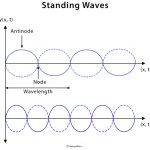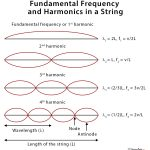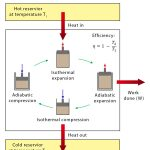Home / Physics / Simple Pendulum

# Simple Pendulum

## Definition: What is a Simple Pendulum?

A pendulum is a device that is found in wall clocks. It consists of a weight (bob) suspended from a pivot by a string or a very light rod so that it can swing freely. When displaced to an initial angle and released, the pendulum will swing back and forth with a periodic motion. By applying Newton’s second law of motion for rotational systems, the equation of motion for the pendulum may be obtained.

Although the pendulum has a long history, Italian scientist Galileo Galilei was the first to study the properties of pendulums, beginning around 1602.

## Terms Associated with Simple Pendulum

Length (L): Distance between the point of suspension to the center of the bob

Time period (T): Time taken by the pendulum to finish one full oscillation

Displacement (x): Distance traveled by the pendulum bob from the equilibrium position to one side. The angle described by the pendulum with an imaginary axis at the equilibrium position is called the angular displacement (θ).

Amplitude (xmax): Maximum distance traveled by the pendulum from the equilibrium position to one side before changing its direction. For angle, it is denoted by θmax.

## Equation of Simple Pendulum

### How to Derive the Formula for Time Period?

According to Newton’s second law,

F = ma

Or, mL d2θ/dt2 = – mg sin θ

Or, d2θ/dt2 + g/L sin θ = 0

If the amplitude of displacement is small, then small-angle approximation holds, i.e., sin θ ~ θ.

d2θ/dt2 + (g/L) θ = 0

Or, d2θ/dt2 + ω2θ = 0

This equation represents a simple harmonic motion. Thus, the motion of a simple pendulum is a simple harmonic motion with an angular frequency, ω = (g/L)1/2 and linear frequency, f = (1/2π) (g/L)1/2. The time period is given by,

T = 1/f = 2π(L/g)1/2.

Starting with the right-hand side, the dimension analysis of the time period gives the unit of time.

[L/LT-2]1/2 = [T]

The principle of a simple pendulum can be understood as follows. The restoring force of the pendulum from the above is, F = -mgL θ. This force is responsible for restoring the pendulum to its equilibrium position. However, due to the inertia of motion, the pendulum passes the equilibrium position and swings to the other side. This motion is periodic and can be solved using differential equation analysis.

After solving the differential equation, the angular displacement is given by

θ = θmax sin (ωt)

Sometimes, a phase φ is added to the above equation depending upon the initial conditions of the pendulum. Then, the equation can be written as

θ = θmax sin (ωt + φ)

A simple pendulum is a typical laboratory experiment in many academic curricula. Students are often asked to evaluate the value of the acceleration due to gravity, g, using the equation for the time period of a pendulum. Rearranging the time period equation,

g = 4π2L/T2

Note that the component mg cos θ is balanced by the tension T of the string, i.e., T = mg cos θ.

## Laws of Simple Pendulum

1. Law of mass: The time period is independent of the mass of the bob.
2. Law of length: The time period is directly proportional to the square root of the length.
3. Law of Iscochronism: The time period is independent of the amplitude as long as the amplitude is small.
4. Law of gravity: The time period is inversely proportional to the square root of the acceleration due to gravity at that place.

## Uses and Applications of the Simple Pendulum

• In a pendulum clock, a common household item. Every time the pendulum swings, the clock’s hand advances at a fixed rate, thus giving the time.
• In older seismometers, a pendulum with a stylus at its bottom was connected to a frame. During an earthquake, the frame moves and causes the stylus to form a pattern on a paper.
• In a pendulum gravimeter, a pendulum is used to measure the local gravity.
• Foucault’s pendulum is a device to measure the rotation of the earth.
• In a metronome, a device used by musicians. It emits a click or a light for each beat of a predetermined interval.

## Simple Pendulum AppletStanding WavesFundamental FrequencyKirchhoff’s LawCarnot Engine